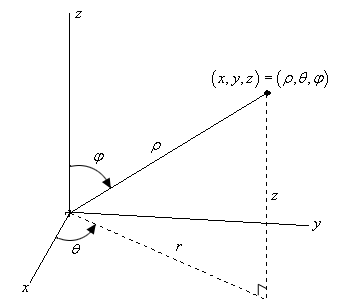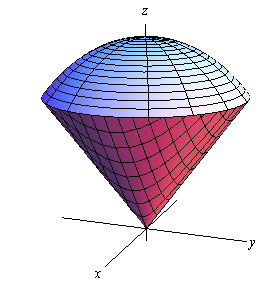Paul's Online Notes
Home / Calculus III / Multiple Integrals / Triple Integrals in Spherical Coordinates
Show Mobile Notice Show All Notes Hide All Notes
Mobile Notice
You appear to be on a device with a "narrow" screen width (i.e. you are probably on a mobile phone). Due to the nature of the mathematics on this site it is best views in landscape mode. If your device is not in landscape mode many of the equations will run off the side of your device (should be able to scroll to see them) and some of the menu items will be cut off due to the narrow screen width.

### Section 15.7 : Triple Integrals in Spherical Coordinates

In the previous section we looked at doing integrals in terms of cylindrical coordinates and we now need to take a quick look at doing integrals in terms of spherical coordinates.

First, we need to recall just how spherical coordinates are defined. The following sketch shows the relationship between the Cartesian and spherical coordinate systems.Here are the conversion formulas for spherical coordinates.

$\begin{array}{c}x = \rho \sin \varphi \cos \theta \hspace{0.25in}y = \rho \sin \varphi \sin \theta \hspace{0.25in}z = \rho \cos \varphi \\ {x^2} + {y^2} + {z^2} = {\rho ^2}\end{array}$

We also have the following restrictions on the coordinates.

$\rho \ge 0\hspace{0.5in}0 \le \varphi \le \pi$

For our integrals we are going to restrict $$E$$ down to a spherical wedge. This will mean that we are going to take ranges for the variables as follows,

$\begin{array}{c}a \le \rho \le b\\ \alpha \le \theta \le \beta \\ \delta \le \varphi \le \gamma \end{array}$

Here is a quick sketch of a spherical wedge in which the lower limit for both $$\rho$$ and $$\varphi$$ are zero for reference purposes. Most of the wedges we’ll be working with will fit into this pattern.From this sketch we can see that $$E$$ is nothing more than the intersection of a sphere and a cone and generally will represent a shape that is reminiscent of an ice cream cone.

In the next section we will show that

$dV = {\rho ^2}\sin \varphi \,d\rho \,d\theta \,d\varphi$

Therefore, the integral will become,

$\iiint\limits_{E}{{f\left( {x,y,z} \right)\,dV}} = \int_{{\,\,\delta }}^{{\,\gamma }}{{\int_{{\,\alpha }}^{{\,\beta }}{{\int_{{\,a}}^{b}{{{\rho ^2}\sin \varphi \,\,f\left( {\rho \sin \varphi \cos \theta ,\rho \sin \varphi \sin \theta ,\rho \cos \varphi } \right)\,d\rho }}\,d\theta }}\,d\varphi }}$

This looks bad but given that the limits are all constants the integrals here tend to not be too bad.

Example 1 Evaluate $$\displaystyle \iiint\limits_{E}{{16z\,dV}}$$ where $$E$$ is the upper half of the sphere $${x^2} + {y^2} + {z^2} = 1$$.
Show Solution

Since we are taking the upper half of the sphere the limits for the variables are,

$\begin{array}{c}0 \le \rho \le 1\\ 0 \le \theta \le 2\pi \\ \displaystyle 0 \le \varphi \le \frac{\pi }{2}\end{array}$

The integral is then,

\begin{align*}\iiint\limits_{E}{{16z\,dV}} & = \int_{0}^{{\,\frac{\pi }{2}}}{{\int_{{\,0}}^{{\,2\pi }}{{\int_{{\,\,0}}^{1}{{{\rho ^2}\sin \varphi \left( {16\rho \cos \varphi } \right)\,d\rho }}\,d\theta }}\,d\varphi }}\\ & = \int_{{\,0}}^{{\,\frac{\pi }{2}}}{{\int_{{\,0}}^{{\,2\pi }}{{\int_{{\,\,0}}^{{\,1}}{{8{\rho ^3}\sin \left( {2\varphi } \right)\,d\rho }}\,d\theta }}\,d\varphi }}\\ & = \int_{{\,0}}^{{\,\frac{\pi }{2}}}{{\int_{{\,0}}^{{\,2\pi }}{{2\sin \left( {2\varphi } \right)\,d\theta }}\,d\varphi }}\\ & = \int_{{\,0}}^{{\,\frac{\pi }{2}}}{{4\pi \sin \left( {2\varphi } \right)\,d\varphi }}\\ & = \left. { - 2\pi \cos \left( {2\varphi } \right)} \right|_0^{\frac{\pi }{2}}\\ & = 4\pi \end{align*}
Example 2 Evaluate $$\displaystyle \iiint\limits_{E}{{z\,x\,dV}}$$ where $$E$$ is inside both $${x^2} + {y^2} + {z^2} = 4$$ and the cone (pointing upward) that makes an angle of $$\frac{\pi }{3}$$ with the negative $$z$$-axis and has $$x \le 0$$.
Show Solution

First, we need to take care of the limits. The region $$E$$ is basically an upside down ice cream cone that has been cut in half so that only the portion with $$x \le 0$$ remains. Therefore, because we are inside a portion of a sphere of radius 2 we must have,

$0 \le \rho \le 2$

For $$\varphi$$ we need to be careful. The problem statement says that the cone makes an angle of $$\frac{\pi }{3}$$ with the negative $$z$$-axis. However, remember that $$\varphi$$ is measured from the positive $$z$$-axis. Therefore, the first angle, as measured from the positive $$z$$-axis, that will “start” the cone will be $$\varphi = \frac{{2\pi }}{3}$$ and it goes to the negative $$z$$-axis. Therefore, we get the following limits for $\varphi$.

$\frac{{2\pi }}{3} \le \varphi \le \pi$

Finally, for the $$\theta$$ we can use the fact that we are also told that $$x \le 0$$. This means we are to the left of the $$y$$-axis and so the range of $$\theta$$ must be,

$\frac{\pi }{2} \le \theta \le \frac{{3\pi }}{2}$

Now that we have the limits we can evaluate the integral.

\begin{align*}\iiint\limits_{E}{{z\,x\,dV}} & = \int_{{\frac{{2\pi }}{3}}}^{{\,\pi }}{{\int_{{\,\frac{\pi }{2}}}^{{\frac{{3\pi }}{2}}}{{\int_{{\,\,0}}^{2}{{\left( {\rho \cos \varphi } \right)\left( {\rho \sin \varphi \cos \theta } \right){\rho ^2}\sin \varphi \,d\rho }}\,d\theta }}\,d\varphi }}\\ & = \int_{{\frac{{2\pi }}{3}}}^{{\,\pi }}{{\int_{{\,\frac{\pi }{2}}}^{{\frac{{3\pi }}{2}}}{{\int_{{\,\,0}}^{2}{{{\rho ^4}\cos \varphi {{\sin }^2}\varphi \cos \theta \,d\rho }}\,d\theta }}\,d\varphi }}\\ & = \int_{{\frac{{2\pi }}{3}}}^{\pi }{{\int_{{\frac{\pi }{2}}}^{{\frac{{3\pi }}{2}}}{{\frac{{32}}{5}\cos \varphi {{\sin }^2}\varphi \cos \theta \,d\theta }}\,d\varphi }}\\ & = \int_{{\frac{{2\pi }}{3}}}^{\pi }{{ - \frac{{64}}{5}\cos \varphi {{\sin }^2}\varphi \,d\varphi }}\\ & = \left. { - \frac{{64}}{{15}}{{\sin }^3}\varphi } \right|_{\frac{{2\pi }}{3}}^\pi \\ & = \frac{{8\sqrt 3 }}{5}\end{align*}
Example 3 Convert $$\displaystyle \int_{{\,0}}^{{\,3}}{{\int_{{\,0}}^{{\sqrt {9 - {y^2}} }}{{\int_{{\sqrt {{x^2} + {y^2}} }}^{{\sqrt {18 - {x^2} - {y^2}} }}{{{x^2} + {y^2} + {z^2}\,dz}}\,dx}}\,dy}}$$ into spherical coordinates.
Show Solution

Let’s first write down the limits for the variables.

$\begin{array}{c}0 \le y \le 3\\ 0 \le x \le \sqrt {9 - {y^2}} \\ \sqrt {{x^2} + {y^2}} \le z \le \sqrt {18 - {x^2} - {y^2}} \end{array}$

The range for $$x$$ tells us that we have a portion of the right half of a disk of radius 3 centered at the origin. Since we are restricting $$y$$’s to positive values it looks like we will have the quarter disk in the first quadrant. Therefore, since $$D$$ is in the first quadrant the region, $$E$$, must be in the first octant and this in turn tells us that we have the following range for $$\theta$$ (since this is the angle around the $$z$$-axis).

$0 \le \theta \le \frac{\pi }{2}$

Now, let’s see what the range for $$z$$ tells us. The lower bound, $$z = \sqrt {{x^2} + {y^2}}$$, is the upper half of a cone. At this point we don’t need this quite yet, but we will later. The upper bound, $$z = \sqrt {18 - {x^2} - {y^2}}$$, is the upper half of the sphere,

${x^2} + {y^2} + {z^2} = 18$

and so from this we now have the following range for $$\rho$$

$0 \le \rho \le \sqrt {18} = 3\sqrt 2$

Now all that we need is the range for $$\varphi$$. There are two ways to get this. One is from where the cone and the sphere intersect. Plugging in the equation for the cone into the sphere gives,

\begin{align*}{\left( {\sqrt {{x^2} + {y^2}} } \right)^2} + {z^2} & = 18\\ {z^2} + {z^2} & = 18\\ {z^2} & = 9\\ z & = 3\end{align*}

Note that we can assume $$z$$ is positive here since we know that we have the upper half of the cone and/or sphere. Finally, plug this into the conversion for $$z$$ and take advantage of the fact that we know that $$\rho = 3\sqrt 2$$ since we are intersecting on the sphere. This gives,

\begin{align*}\rho \cos \varphi & = 3\\ 3\sqrt 2 \cos \varphi & = 3\\ \cos \varphi = \frac{1}{{\sqrt 2 }} & = \frac{{\sqrt 2 }}{2}\hspace{0.5in} \Rightarrow \hspace{0.5in}\varphi = \frac{\pi }{4}\end{align*}

So, it looks like we have the following range,

$0 \le \varphi \le \frac{\pi }{4}$

The other way to get this range is from the cone by itself. By first converting the equation into cylindrical coordinates and then into spherical coordinates we get the following,

\begin{align*}z& = r\\ \rho \cos \varphi & = \rho \sin \varphi \\ 1 & = \tan \varphi \hspace{0.5in} \Rightarrow \hspace{0.5in}\varphi = \frac{\pi }{4}\end{align*}

So, recalling that $${\rho ^2} = {x^2} + {y^2} + {z^2}$$, the integral is then,

$\int_{{\,0}}^{{\,3}}{{\int_{{\,0}}^{{\sqrt {9 - {y^2}} }}{{\int_{{\sqrt {{x^2} + {y^2}} }}^{{\sqrt {18 - {x^2} - {y^2}} }}{{{x^2} + {y^2} + {z^2}\,dz}}\,dx}}\,dy}} = \int_{{\,0}}^{{\,{\pi }/{4}\;}}{{\int_{{\,0}}^{{{\pi }/{2}\;}}{{\int_{{\,0}}^{{\,3\sqrt 2 }}{{{\rho ^4}\sin \varphi \,d\rho }}\,d\theta }}\,d\varphi }}$